• 基于 matlab 的 floyd 算法 matlab 计算最短路径 function [d,path]=floyd(a,sp,ep) % floyd - 最短路问题 % % Syntax: [d,path]=floyd(a,sp,ep) % % Inputs: % a - 距离矩阵是指 i 到 j 之间的距离可以是有向的 % ...
• 最近老板让计算最短路径及路径个数，找遍了所有工具箱，都没现成的。急死了，什么Dijkstra和Floyd都搞不定。最后，想了想，算了吧，自己编吧，反正自己用，又没有算法复杂度要求。于是自己就写了个小程序（本程序仅...
    最近老板让计算最短路径及路径个数，找遍了所有工具箱，都没现成的。急死了，什么Dijkstra和Floyd都搞不定。最后，想了想，算了吧，自己编吧，反正自己用，又没有算法复杂度要求。于是自己就写了个小程序（本程序仅限无权无向连通图），算法复杂度不晓得（）。
本人不是计算机出身，就不写算法步骤了，直接上图解。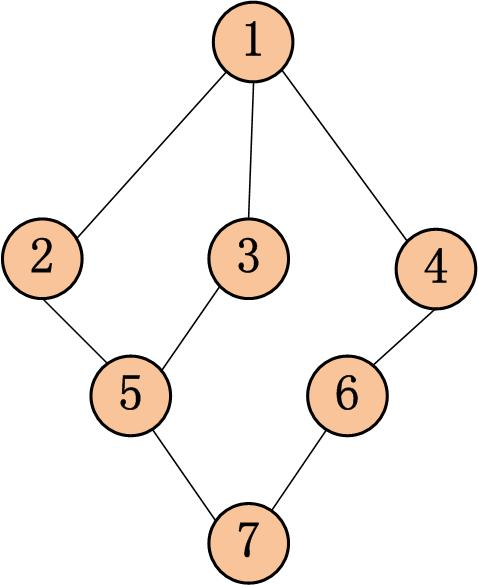我们首先计算的是节点1到所有节点的最短路径，及个数。s存放节点1到所有节点的最短路径，p_num存放路径的个数。
初始化：s=[0,0,0,0,0,0,0]      p_num=[1,0,0,0,0,0,0]
第一轮迭代：找节点1的所有邻居节点2,3,4。则：s（[2,3,4]）=1
在看节点2的邻居1和5.则： p_num（2）= p_num（1）+ p_num（5）=1+0=1
同理：p_num（3）=p_num（4）=1
则本轮会得到：s=[0,1,1,1,0,0,0]      p_num=[1,1,1,1,0,0,0]

第二轮迭代：找节点2,3,4的所有邻居节点（没有被找过的节点）5,6。则：s（[5,6]）=2
再看节点5的邻居2,3和7.则： p_num（5）= p_num（2）+ p_num（3）+ p_num（7）=1+1+0=2
同理：p_num（6）=1
则本轮会得到：s=[0,1,1,1,2,2,0]      p_num=[1,1,1,1,2,1,0]
第三轮迭代：找节点5,6的所有邻居节点（没有被找过的节点）7。则：s（）=3
在看节点7的邻居5和6.则： p_num（2）= p_num（5）+ p_num（6）=2+1=3
则本轮会得到：s=[0,1,1,1,2,2,3]      p_num=[1,1,1,1,2,1,3]
找节点7的所有邻居节点（没有被找过的节点），没啦，迭代终止。哈哈节点到所有节点的最短路径及条数搞定，然后来个循环就把任意两个点的最短路径及个数搞定。
哇嘎嘎，简单粗暴，下面有程序，能力有限。使用前记得要仔细拍错误哦哦

function [all_s,all_p_num]=all_node_shortest(w)
%全联通无权无向图
%输入邻接矩阵w
%all_s为所有节点间的最短距离
%all_p_num为最短路径的个数
%树状搜索（纯属自己想的,没有优化，可以跑小网络）
n=length(w);                    %节点的个数
all_s=zeros(n,n);               %初始化
all_p_num=zeros(n,n);       %初始化
for i=1:n
[s,p_num]=node_shortest(w,i);
all_s(i,:)=s;
all_p_num(i,:)=p_num;
end
end
function [s,p_num]=node_shortest(w,node)
%全联通无权无向图
%输入邻接矩阵w
%初始节点 node
%s为node到所有节点的最短距离
%p_num为最短路径的个数
%树状搜索（纯属自己想的）
n=length(w);                    %节点的个数
s=zeros(1,n);                   %初始化
p_num=zeros(1,n);               %初始化
p_num(node)=1;                %node到node本身距离为零，个数记为1
node_all=zeros(1,n);
node_all(node)=1;               %记录哪些节点被搜索到，搜索到的节点记为1，否则为0
lj=node;                        %lj为当前步到达的节点（到lj的距离都为k）
k=1;
while sum(node_all)<n           %搜索至所有节点停止迭代
lj=find(sum(w(lj,:),1)>0&node_all==0);      %上一时刻的所有节点的邻居且没有被找到过的节点是这一次的邻居
s(lj)=k;                                    %记录时刻即路径长度
p_num(lj)=sum(w(:,lj).*repmat(p_num',[1,length(lj)]),1); %这一时刻的路径的条数为：这个节点上一时刻已经到达的邻居的所有路径和
node_all(lj)=1;                                          %这些节点在这个时刻已经被找到
k=k+1;                                                   %下一时刻
end
end
展开全文• floyd算法+matlab计算，对数学建模爱好者有很大的好处~~~~
• 利用 Matlab 编程计算最短路径及中位点选址，最短路问题 两个指定顶点之间的最短路径。
• 基于MATLAB最短路径SPFA算法 前面已述 对应单一源点到各顶点的最短路径算法 贝尔曼-福特和迪杰斯拉特算法 现在说明一下SPFA算法精髓： 在之前的Bellman-Ford算法中存在一个重复更新的问题，即每个枢纽点都要对全部...
基于MATLAB的最短路径SPFA算法
前面已述 对应单一源点到各顶点的最短路径算法 贝尔曼-福特和迪杰斯拉特算法
现在说明一下SPFA算法精髓：
在之前的Bellman-Ford算法中存在一个重复更新的问题，即每个枢纽点都要对全部顶点更新计算
SPFA算法就解决了重复计算的问题，在大数据面前大大减少运行时间
该算法改善的思想是避免顶点进行无效的重复更新，对有待更新的顶点移入队列，已更新的顶点移出队列，避免待更新的顶点中存在重复顶点
现通过MATLAB软件阐述算法程序和运行结果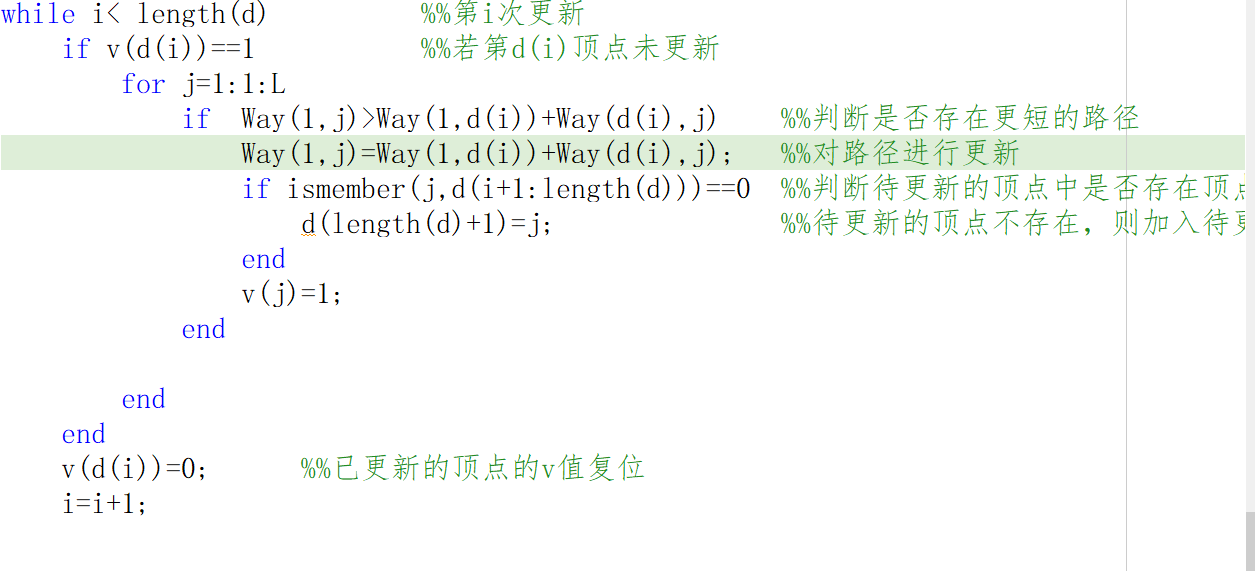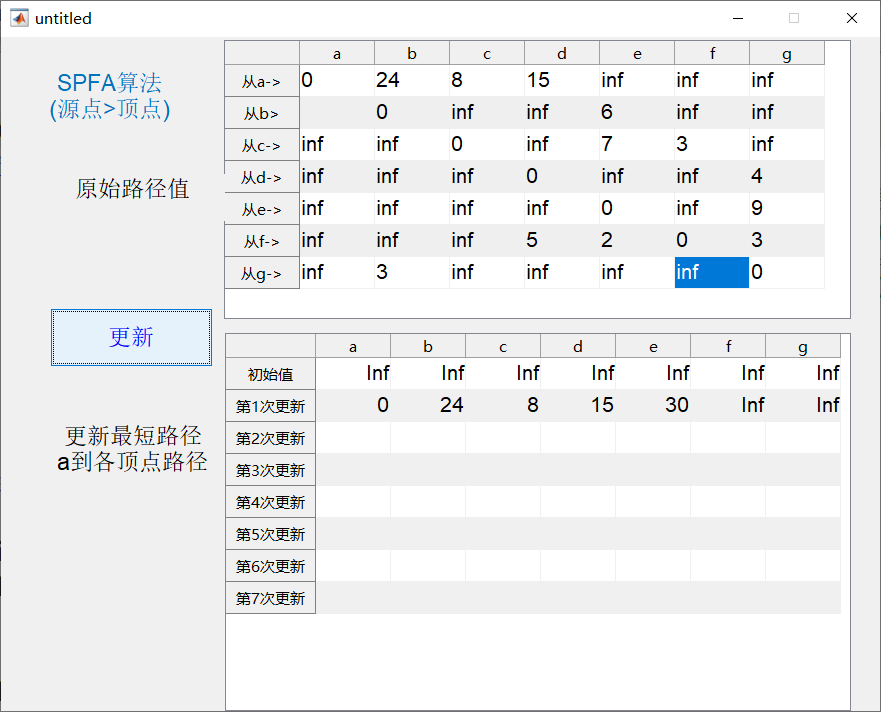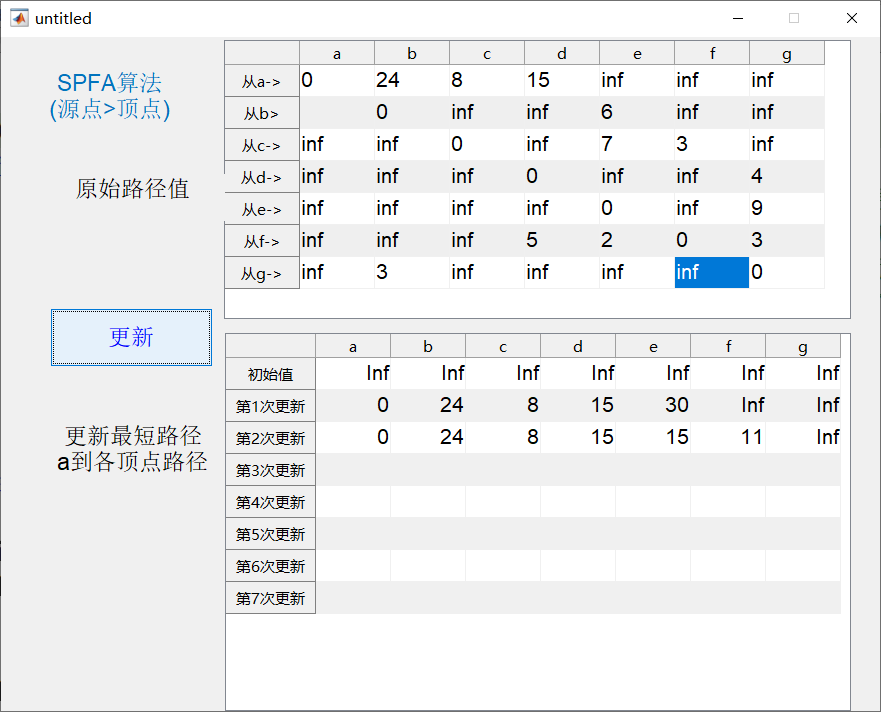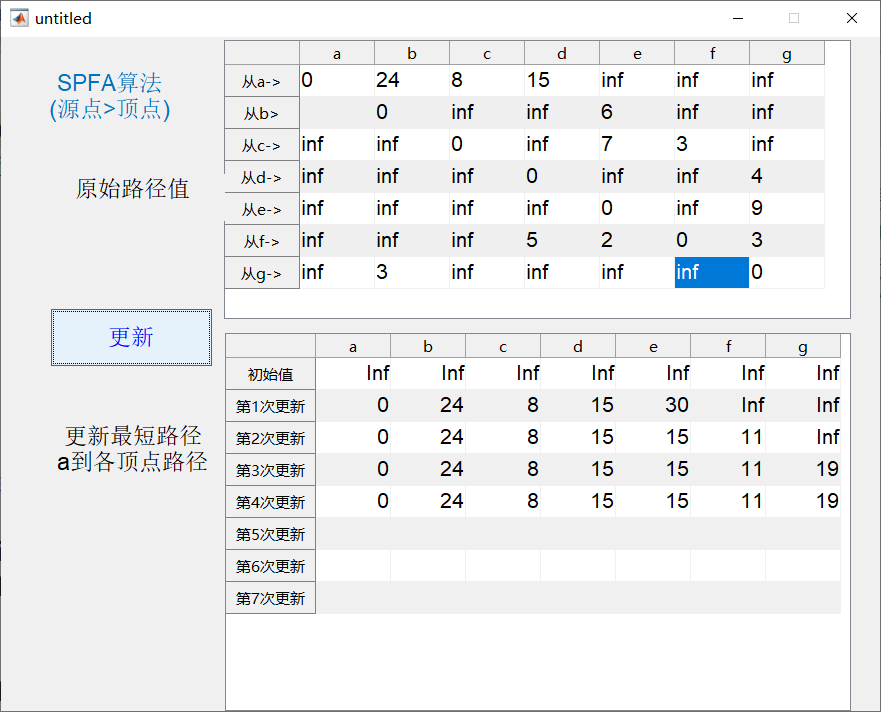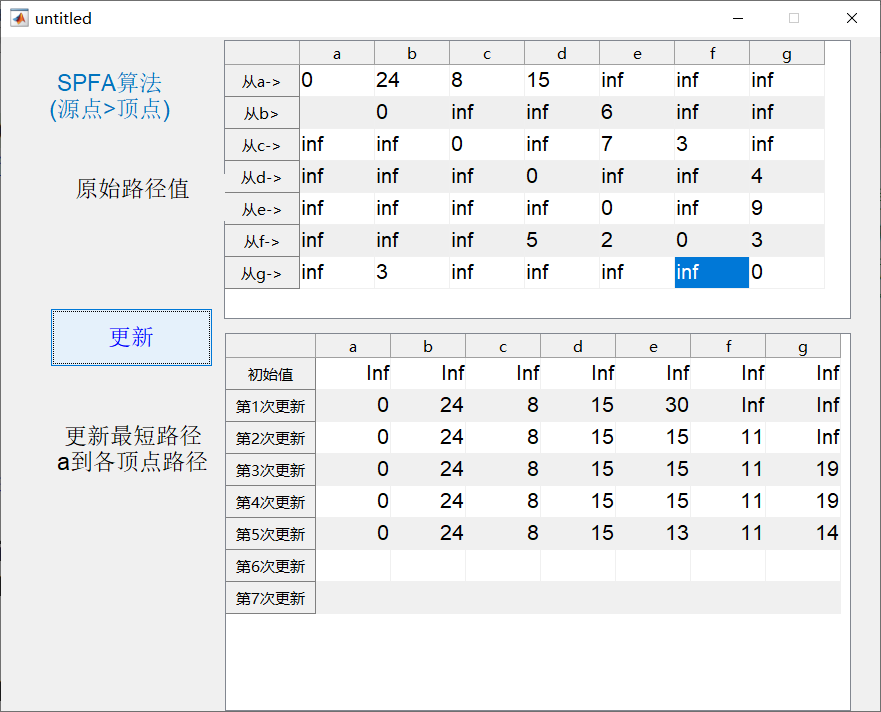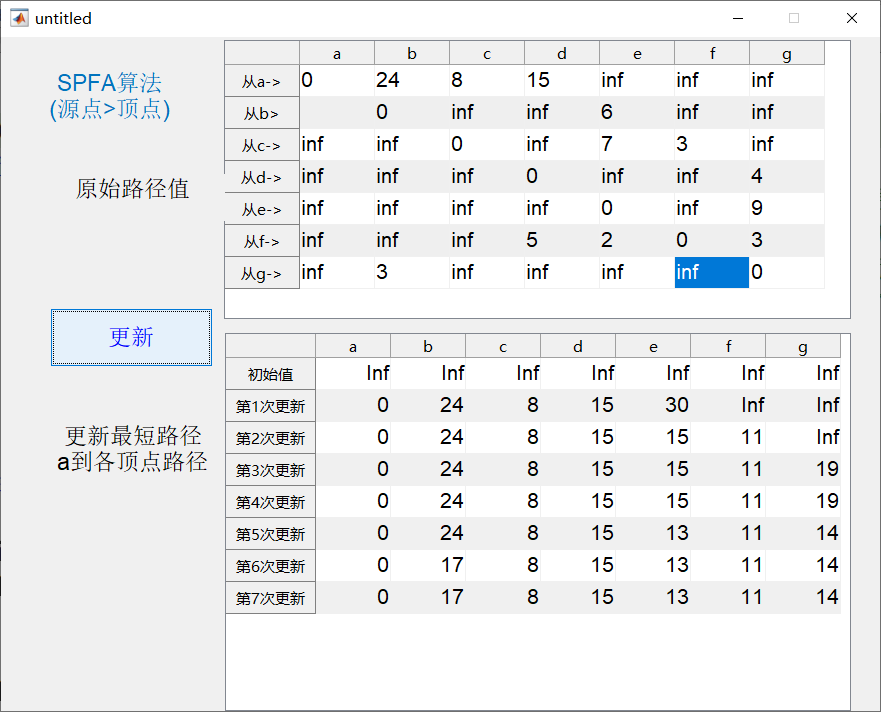展开全文算法
• 图论最短路径 （1）matlab作图 G = graph(s, t) 其中，s 和 t 都是节点组成的向量，表示从s 、t对应节点之间有边。 然后，plot(G)即可画出图。 G = graph(s, t, w) ...（2）Matlab计算最短路径 [P,d] = s
图论最短路径
（1）matlab作图
G = graph(s, t)
其中，s 和 t 都是节点组成的向量，表示从s 、t对应节点之间有边。
然后，plot(G)即可画出图。
G = graph(s, t, w)
其中，w表示对应边的权重。
例如：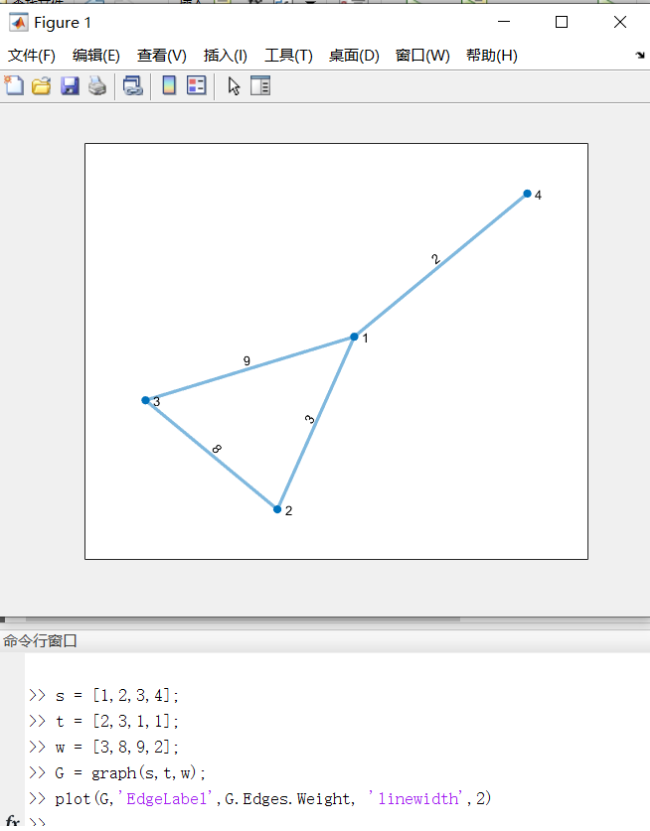这里用 EdgeLabel 属性把权重设置到边上，设置linewidth 属性为2来改变线宽。
有向图把 graph 改成 digraph 即可。
注意：matlab编号是从1开始连续编号，所以编号时要注意。
（2）Matlab计算最短路径
[P,d] = shortestpath(G,start,end [,‘Method’,‘algorithm’])
其中，
（1）G是一个图（graph对象 | digraph对象）
（2）start是起始节点，end目标节点。
（3）后面是可选参数，一般不用调，默认是auto。可选值：auto、unweighted（无权图）、positive（Dijkstra算法，要求权重非负）、mixed（允许权重为负）。
（4）P是最短路径经过的节点向量
（5）d是最短距离。
例如：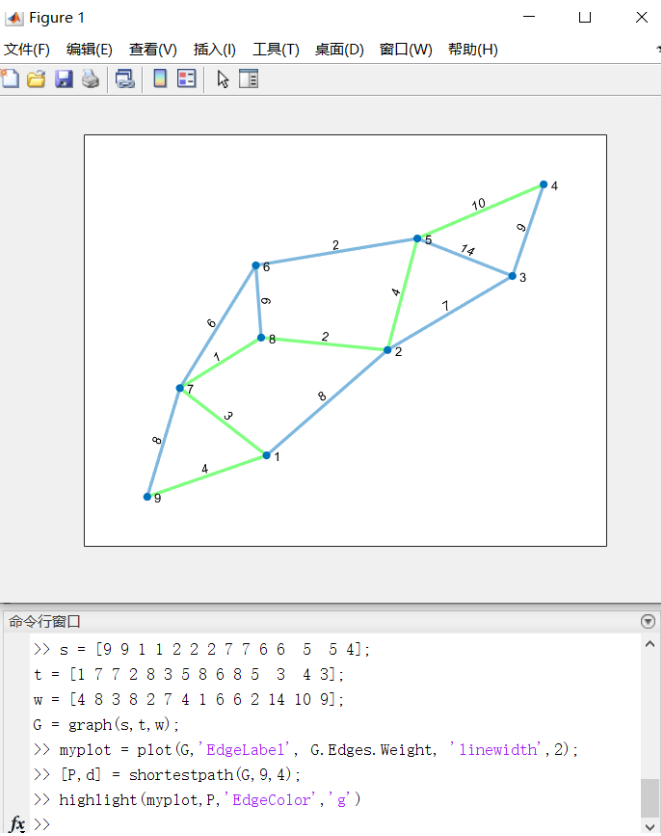这里把最短路径高亮了。
（3）求任意两点的距离矩阵
d = distances(G [,‘Method’, algorithm])
其中
（1）G是一个图。（graph对象 | digraph对象）
（2）返回值 d 是一个方阵，表示两两之间的最短距离。
（4）找给定范围内所有的点
[nodeIDs, dist] = nearest(G,s,d, [‘Method’, algorithm])
表示返回图 G 中距离 s 节点在d 之内的所有结点及距离。
其中，nodeIDs是结点向量、dist是距离向量。


展开全文• 用遗传算法计算最短路径MATLAB程序，在数学建模及其他编程中一种重要的算法思想
• matlab实现的，用遗传算法计算最短路径，都有源码，有需要的朋友可以分享下，很不错哦！
• MATLAB实现的最短路径算法，在图论里比较重要，可以计算出个对象之间的距离。
• 复杂网络最短路径代码，可以学习，可以直接用。能很好的计算出网络的最短路径
• 网络节点间最短路径长度计算matlab程序，采用弗洛伊德算法
• Matlab最短路径算法程序，可用于计算一个起点至一个终点或多个终点的最短距离及行驶路径。
• 图论基本概念及如何作图 无向图的权重邻接矩阵 有向图的权重邻接矩阵 狄杰斯特拉算法和贝尔曼福特算法求解最短路径 狄杰斯特拉算法 模板： ...visited：是否访问过；...matlab计算最短路径： [P,d] = shortestpath(G
图论基本概念及如何作图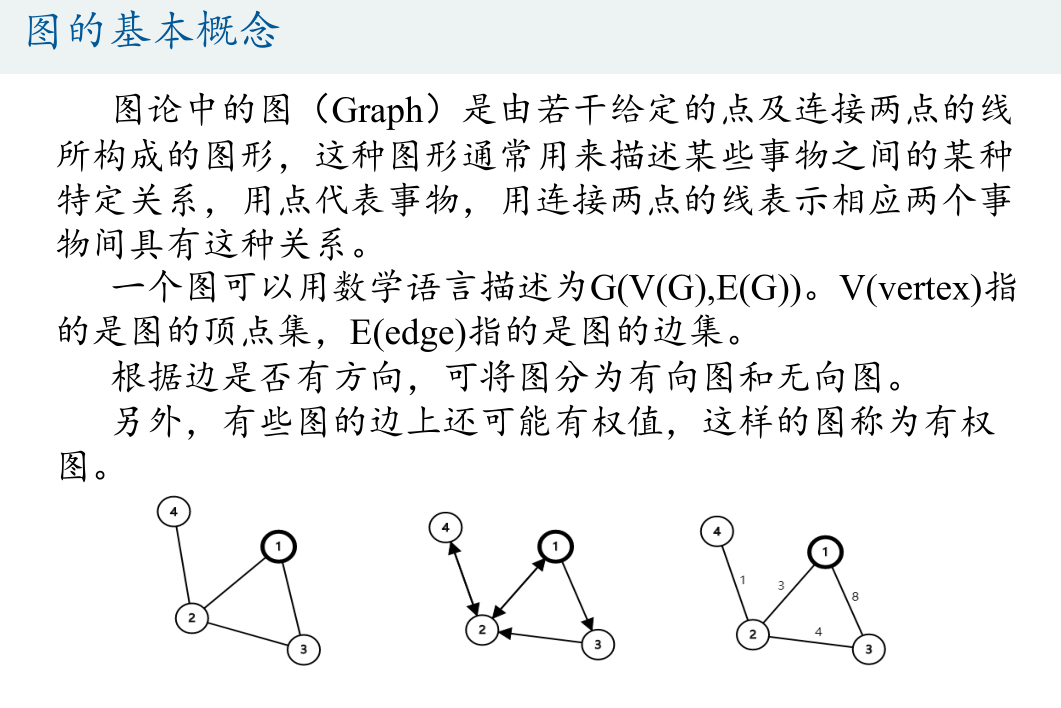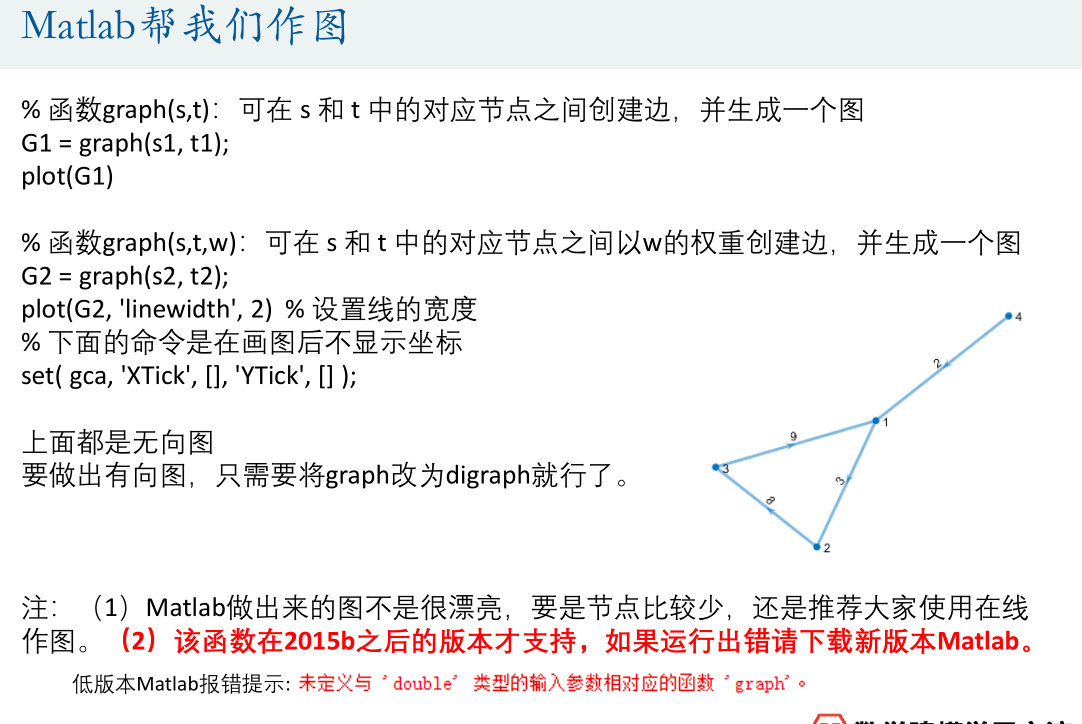无向图的权重邻接矩阵有向图的权重邻接矩阵狄杰斯特拉算法和贝尔曼福特算法求解最短路径
狄杰斯特拉算法
模板：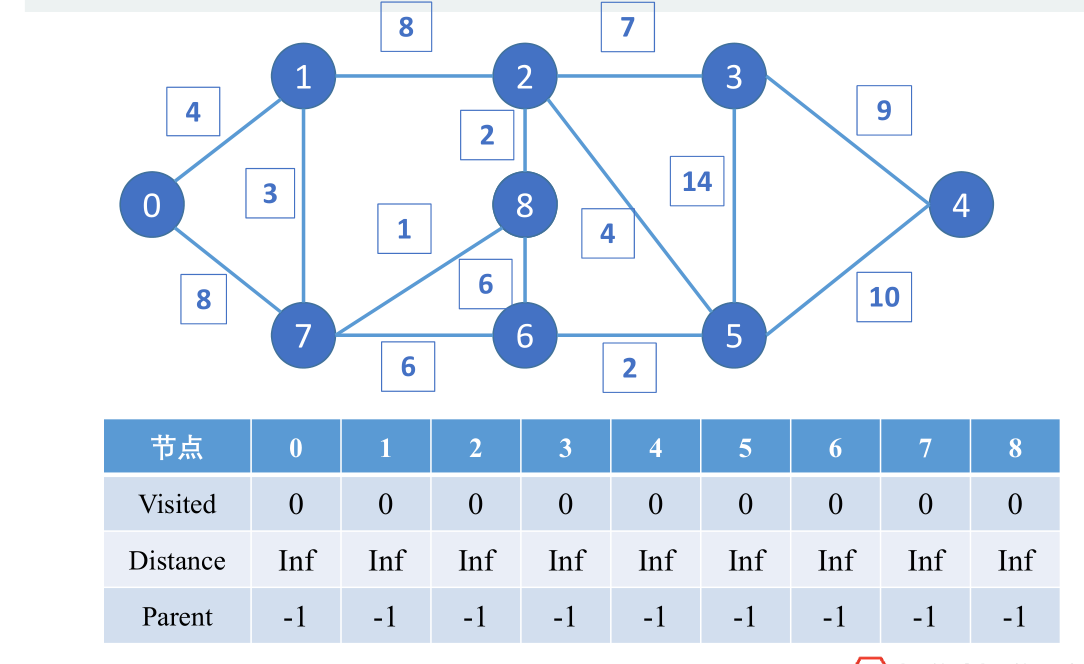visited：是否访问过；
distance：最短距离；
parent：上一节点；
主要思想：
确定起点后寻找下一联接点，不断更新最短路径，以未访问过点的最短路径为下一联接点，继续更新，循环直至到终点；
缺点：
不能处理负权重问题；
解决方法：贝尔曼福特算法
贝尔曼福特算法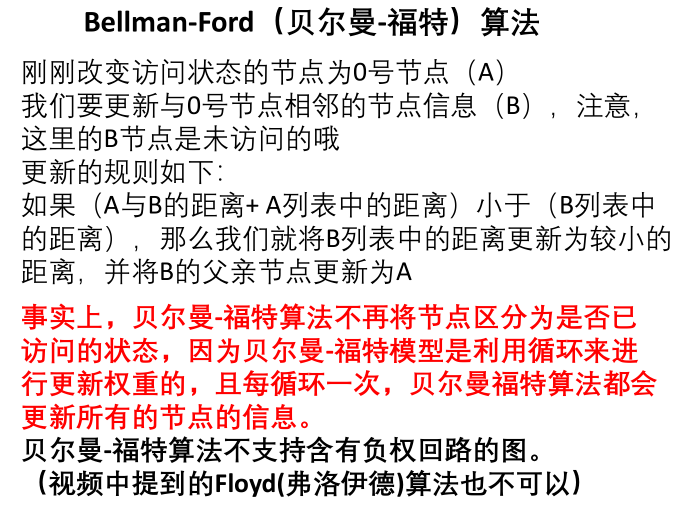负权回路：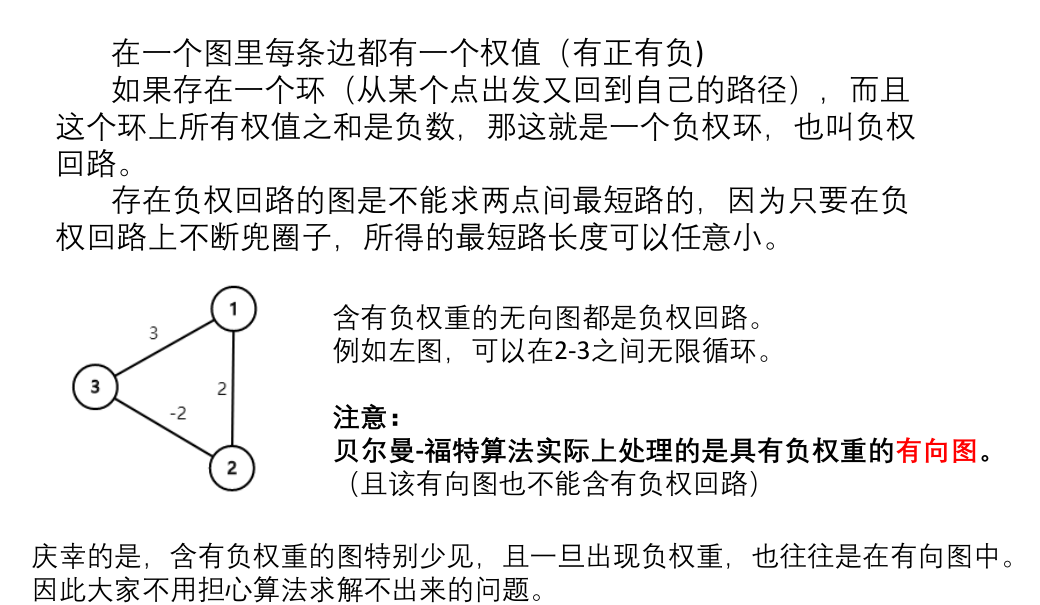matlab计算最短路径：
[P,d] = shortestpath(G,start,end [,‘Method’,algorithm] )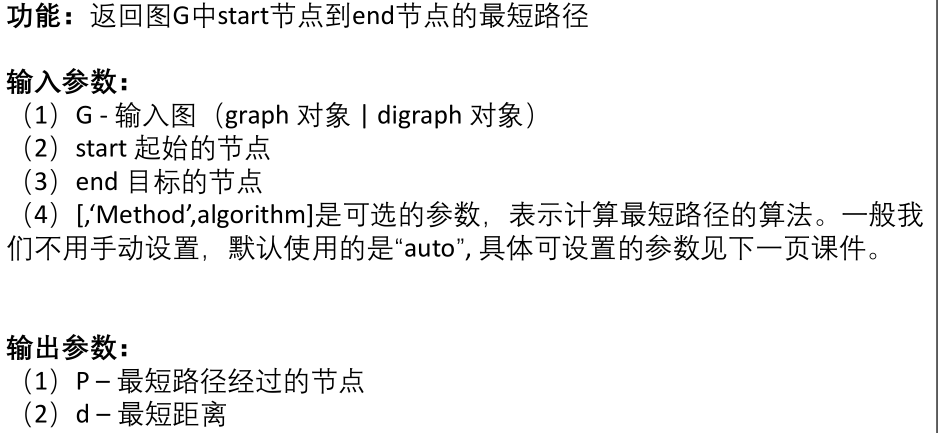可选的算法：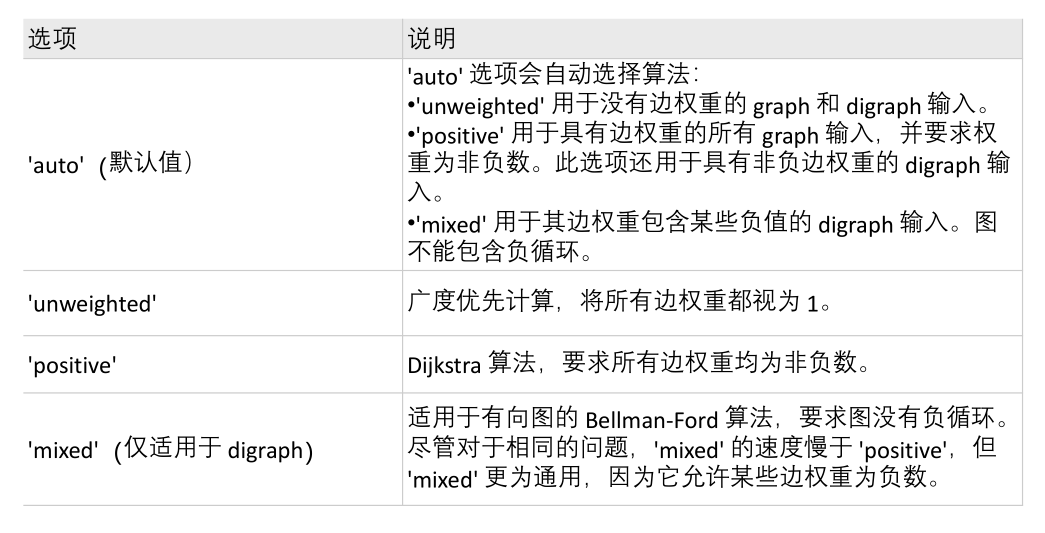PS:
matlab中的图节点要从1开始编；
编号最好是从1开始连续编号，不要自己随便定义编号；
返回任意两点的距离矩阵
d = distances(G [,‘Method’,algorithm])
找给定范围内所有的点
[nodeIDs,dist] = nearest(G,s,d [,‘Method’,algorithm])
返回图形G中与节点s的距离在d之内的所有节点，nodeIDs是符合条件的节点，Dist是这些节点与s的距离。


展开全文数学建模
• 第八讲：图论最短路径问题 图的基本概念 在线做图 Matlab帮我们作图 无向图的权重邻接矩阵 ...Matlab计算最短路径 可选的算法 Matlab演示 返回任意两点的距离矩阵 找给定范围内所有的点 课后作业 ...算法 数学建模
• % 利用dijkstra算法计算两点间的最短路径 % A：邻接矩阵 % strat：起点编号 % dest：终点编号 % path：最短路径索引 % distence：最短路径下的距离值 function [dist,path] = dijkstra(A,start,dest) % 测试数据 A =...
• 旅行商问题 遗传算法 C++求解 MATLAB拟合 加权求最优
• 蚁群算法，MATLAB实现，可以实现最小路径计算
• 我们要计算从源到所有其他各顶点的最短路径长度。这里的长度是指路上各边权之和。这个问题通常称为单源最短路径问题。 实例设计： 已知量： 源节点：红方块位置 通路：蓝点相连表示 目标节点：绿色方块位置 求解量...
• A *搜索算法是一种简单有效的技术，可用于计算到达目标位置的最短路径。本代码介绍了该算法的详细说明和一个交互式演示。 代码获取
• 这是一道比较有意思的题目，给定一个数字方阵，你只能向左或者向下，让你... 我们可以把所有可能的路径找出来，然后计算每一条路径的值，然后进行比较，找出最短路径。但我们不需要这么做，毕竟设计一个遍历所有路...
• 内含最短路径算法代码及实验报告。本次实验要求利用MATLAB分别实现Dijkstra算法和Floyd算法，可对输入的邻接距离矩阵计算图中任意两点间的最短距离矩阵和路由矩阵，且能查询任意两点间的最短距离和路由。
•  旅行商问题就是找到经过所有站点的最短闭合路径，如下图为在美国地图框架内产生的200个旅行站点，而旅行商要找到一条最短路径将200个站点都旅行到。这也可以借助二元整数规划求解。  这个例子比较典型：  ...求解
• 是从一个顶点到其余各顶点的最短路径算法，解决的是有权图中最短路径问题。迪杰斯特拉算法主要特点是从起始点开始，采用贪心算法的策略，每次遍历到始点距离最近且未访问过的顶点的邻接节点，直到扩展到终点为止。 ...算法
• 粒子群优化算法(PSO：Particle swarm optimization) 是一种进化计算技术（evolutionary computation）。源于对鸟群捕食的行为研究。粒子群优化算法的基本思想：是通过群体中个体之间的协作和信息共享来寻找最优解． ...
• 针对羊场煤矿,选取巷道节点,根据节点坐标,计算巷道实际长度和当量长度,构建当量长度邻接矩阵,基于蚁群算法和MATLAB仿真平台,得到救援最短路径及距离,并对结果进行优化,确保救援工作遍历所有巷道,给出了实际可行的...
•  基于机器人在平面区域运动的避障问题，通过单一障碍物路径长度设计算法，利用MATLAB软件进行分别计算，综合比较得出机器人从区域起点到达目标点的避障最短路径
• 首先，函数n2shorf用来计算任意两点之间最短路径长度及最短路经过的节点 需输入起点、终点 1 function [ P u] = n2shorf( W,k1,k2) 2 3 %W表示权值矩阵 4 %k1表示始点，k2表示终点 5 %P表示两顶点...
• 粒子群优化算法(PSO：Particle swarm optimization) 是一种进化计算技术（evolutionary computation）。源于对鸟群捕食的行为研究。粒子群优化算法的基本思想：是通过群体中个体之间的协作和信息共享来寻找最优解． ...
• 算法描述： 输入图G，源点v0，输出...2.计算v0到v1各点的最短距离，保存到D for each i in v0;D(j)=min[D(j),G(v0(1),i)+G(i,j)] ,where j in v1 3.将D中最小的那一项加入到v0，并且从v1删除这一项。 4.转到2，...# matlab计算最短路径matlab 订阅